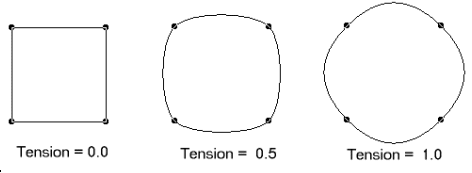﻿ Tension Property (RasterCurve) | Leadtools | Raster, Medical, Document Help
←Select platform
In This Topic ▼

# Tension Property

Summary
Gets or sets a value that indicates how straight to make the curves that connect points.
Syntax
C#
VB
C++
``public double Tension { get; set; } ``
``Public Property Tension As Double ``
````public: `
`property double Tension { `
`   double get(); `
`   void set (    double ); `
`} ````

#### Property Value

An Double value that indicates how straight to make the curves that connect points. Valid values are between 0.0 and 1.0.

Remarks

This value is used only when Type is RasterCurveType.Standard. Valid values are between 0.0 and 1.0, where 0.0 indicates straight lines, and 1.0 indicates lines with the highest curvature. A typical value for dTension is 0.5.Example

For an example, refer to RasterCurve.

Requirements